Anúncio

# Ph ddefence

professor em Polytechnic of Zagreb
1 de Oct de 2013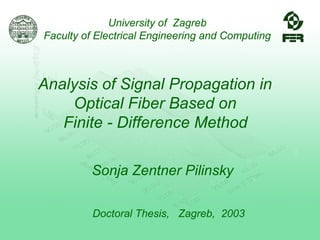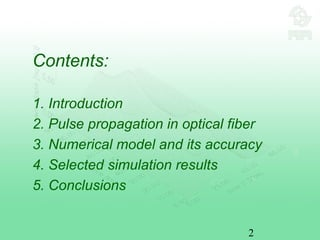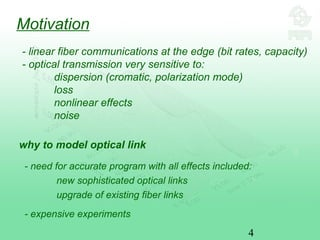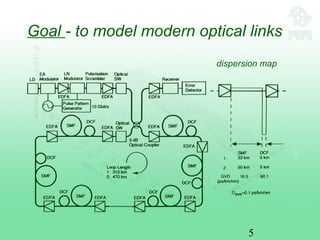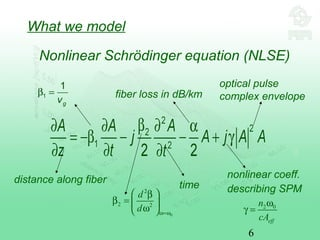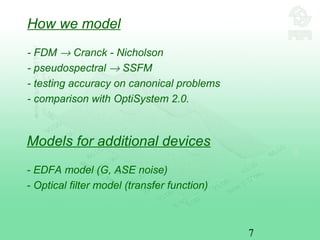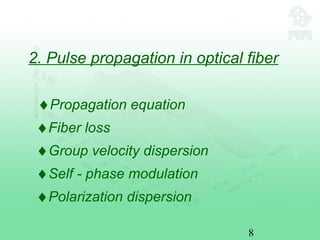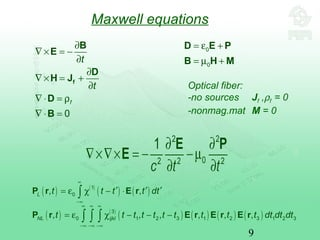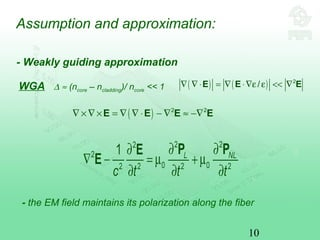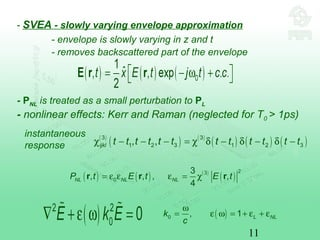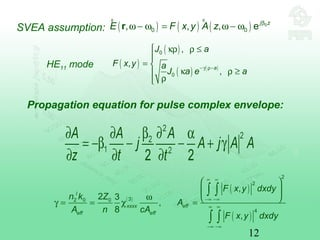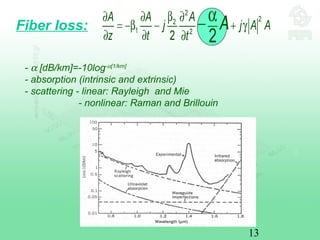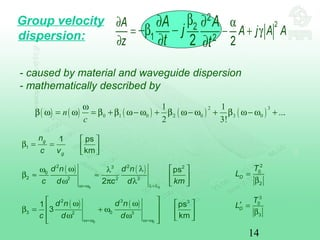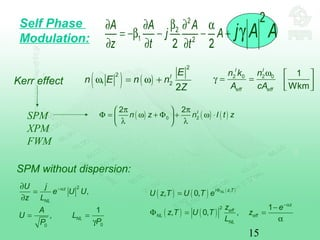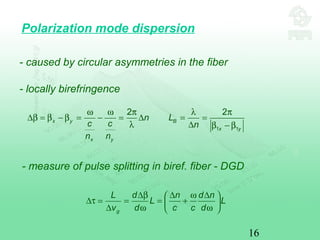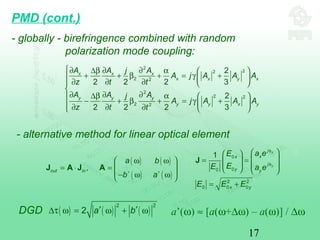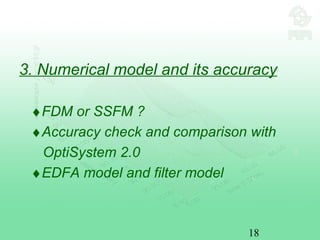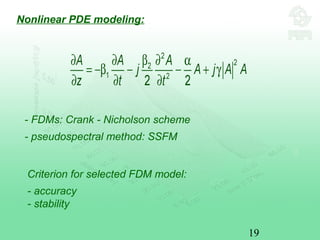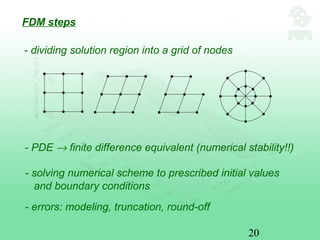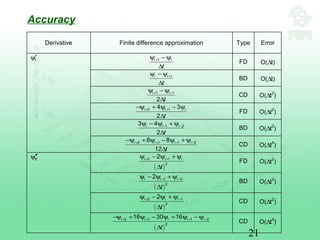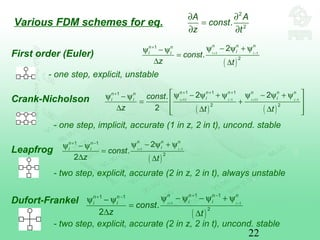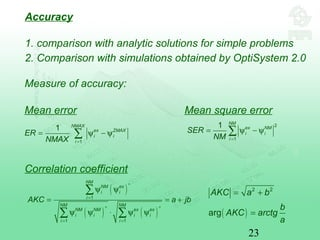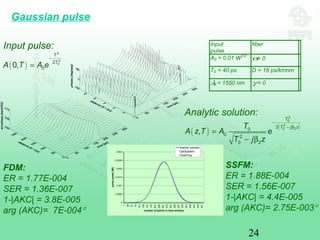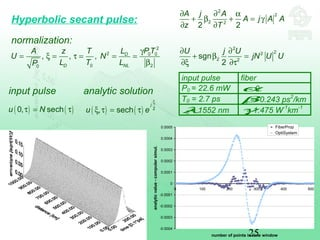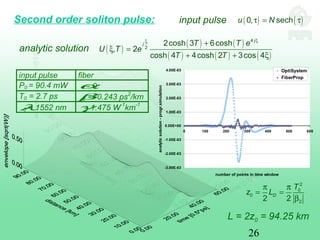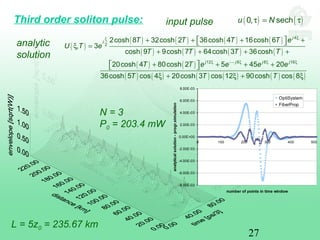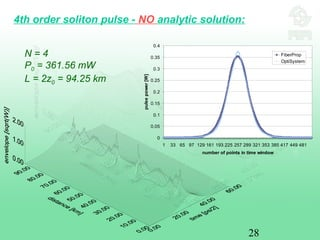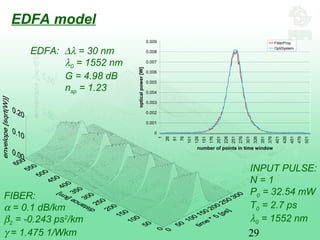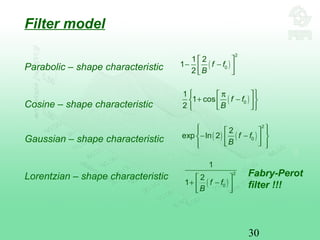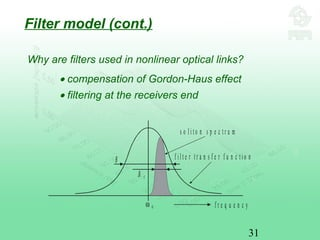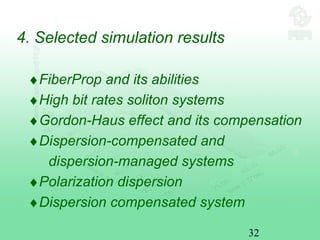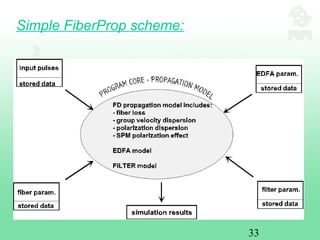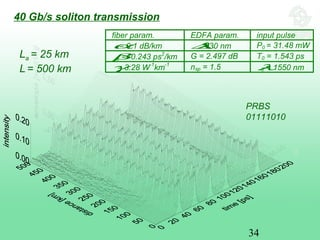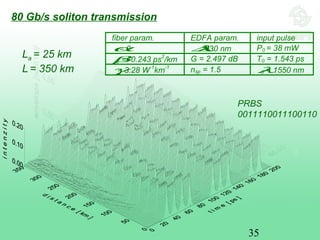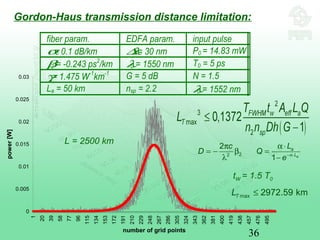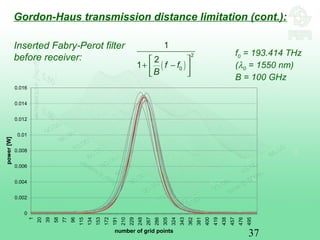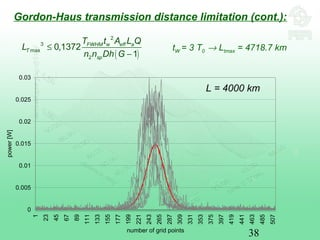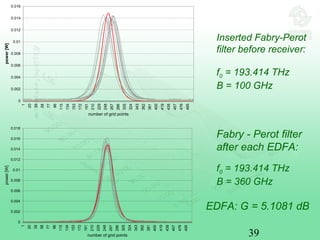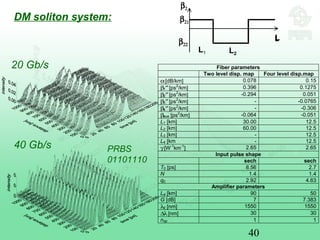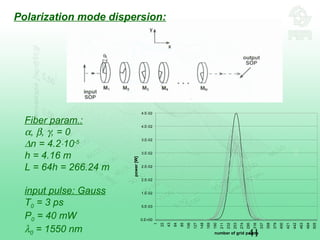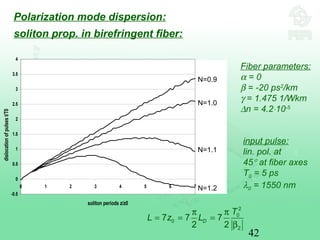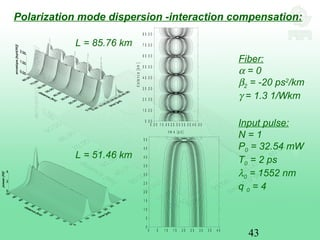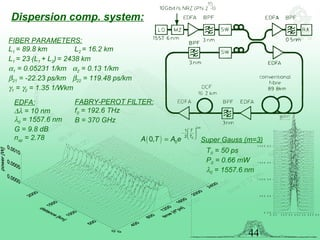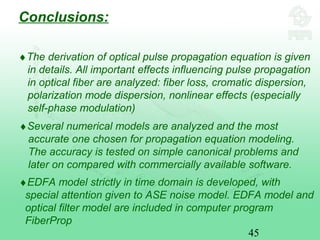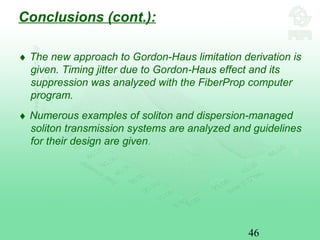1 de 46
Anúncio

### Ph ddefence

1. University of Zagreb Faculty of Electrical Engineering and Computing Analysis of Signal Propagation in Optical Fiber Based on Finite - Difference Method Sonja Zentner Pilinsky Doctoral Thesis, Zagreb, 2003
2. 2 Contents: 1. Introduction 2. Pulse propagation in optical fiber 3. Numerical model and its accuracy 4. Selected simulation results 5. Conclusions
3. 3 1. Introduction ♦Motivation and goal ♦What we model ♦How we model ♦Additional devices needed
4. 4 Motivation - need for accurate program with all effects included: new sophisticated optical links upgrade of existing fiber links - expensive experiments why to model optical link - linear fiber communications at the edge (bit rates, capacity) - optical transmission very sensitive to: dispersion (cromatic, polarization mode) loss nonlinear effects noise
5. 5 Goal - to model modern optical links dispersion map
6. 6 What we model Nonlinear Schrödinger equation (NLSE) 0 2 2 2 ω=ω  β β =   ω  d d nonlinear coeff. describing SPM fiber loss in dB/km time 1 1 gv β = distance along fiber optical pulse complex envelope 2 0ω γ = eff n cA
7. 7 How we model - FDM → Cranck - Nicholson - pseudospectral → SSFM - testing accuracy on canonical problems - comparison with OptiSystem 2.0. Models for additional devices - EDFA model (G, ASE noise) - Optical filter model (transfer function)
8. 8 2. Pulse propagation in optical fiber ♦Propagation equation ♦Fiber loss ♦Group velocity dispersion ♦Self - phase modulation ♦Polarization dispersion
9. 9 Maxwell equations 0 f t t ∂ ∇ × = − ∂ ∂ ∇ × = + ∂ ∇ ⋅ = ρ ∇ ⋅ = f B E D H J D B 0 0 = ε + = µ + D E P B H M Optical fiber: -no sources Jf ,ρf = 0 -nonmag.mat M = 0 ( ) ( ) ( ) ( )1 0, ,L t t t t dt ∝ −∝ ′ ′ ′= ε χ − ⋅∫P r E r ( ) ( ) ( ) ( ) ( ) ( )3 0 1 2 3 1 2 3 1 2 3, , , , , ,NL ijklt t t t t t t t t t dt dt dt ∝ ∝ ∝ −∝ −∝ −∝ = ε χ − − −∫ ∫ ∫P r E r E r E r
10. 10 Assumption and approximation: ( ) ( ) 2 /∇ ∇ ⋅ = ∇ ⋅∇ε ε << ∇E E E ( ) 2 2 ∇ × ∇ × = ∇ ∇ ⋅ − ∇ ≈ −∇E E E E WGA ∆ ≈ (ncore – ncladding)/ ncore << 1 - the EM field maintains its polarization along the fiber - Weakly guiding approximation
11. 11 - PNL is treated as a small perturbation to PL - nonlinear effects: Kerr and Raman (neglected for T0 > 1ps) instantaneous response ( ) ( ) ( ) ( ) ( ) ( )3 3 1 2 3 1 2 3, ,ijkl t t t t t t t t t t t tχ − − − = χ δ − δ − δ − - SVEA - slowly varying envelope approximation - envelope is slowly varying in z and t - removes backscattered part of the envelope ( )0 , 1 L NLk c ω = ε ω = + ε + ε ( ) ( ) ( ) ( ) 23 0 3 , , , , 4 NL NL NLP t E t E t= ε ε ε = χr r r
12. 12 ( ) ( ) ( ) 2 2 32 0 0 4 , 2 3 , 8 , I xxxx eff eff eff F x y dxdy n k Z A A n cA F x y dxdy ∝ ∝ −∝ −∝ ∝ ∝ −∝ −∝     ω  γ = = χ = ∫ ∫ ∫ ∫ Propagation equation for pulse complex envelope: SVEA assumption: ° ( ) ( ) ° ( ) 0 0 0, , , ej z E F x y A z β ω − ω = ω − ωr HE11 mode ( ) ( ) ( ) ( ) 0 0 , , , a J a F x y a J a e a −γ ρ−  κρ ρ ≤  =  κ ρ ≥ ρ
13. 13 - α [dB/km]=-10log-α[1/km] - absorption (intrinsic and extrinsic) - scattering - linear: Rayleigh and Mie - nonlinear: Raman and Brillouin Fiber loss:
14. 14 - caused by material and waveguide dispersion - mathematically described by ( ) ( ) ( ) ( ) ( ) 2 3 0 1 0 2 0 3 0 1 1 .. 2 3! . ω β ω = ω = β +β ω− ω + β ω− ω + β ω− ω +n c 1 1 ps km g g n c v   β = =     ( ) ( ) 0 0 2 23 2 0 2 2 2 2 ps 2 d n d n c d c d kmω=ω λ=λ ω λ  ω λ β ≈ ≈   ω π λ   ( ) ( ) 0 0 2 3 3 3 02 3 1 ps 3 km d n d n c d dω=ω ω=ω  ω ω    β = + ω   ω ω     2 0 2 D T L = β 3 0 3 D T L′ = β Group velocity dispersion:
15. 15 ( ) ( ) 2 2 2, 2 I E n E n n Z ω = ω +Kerr effect SPM XPM FWM 2 0 2 0 1 Wkm I I eff eff n k n A cA ω   γ = =     SPM without dispersion: 2 00 , 1 , z NL NL U j e U U z L A U L PP −α∂ = ∂ = = γ Self Phase Modulation: ( ) ( ) ( )0 2 2 2 I n z n I t z π π  Φ = ω + Φ + ω ⋅  λ λ  ( ) ( ) ( ) ( ) ( ) , 2 , 0, 1 , 0, , NLi z T z eff NL eff NL U z T U T e z e z T U T z L Φ −α = − Φ = = α
16. 16 Polarization mode dispersion - caused by circular asymmetries in the fiber - locally birefringence 2 x y x y n c c n n ω ω π ∆β = β − β = − = ∆ λ 1 1 2 B x y L n λ π = = ∆ β − β - measure of pulse splitting in biref. fiber - DGD g L d n d n L L v d c c d ∆β ∆ ω ∆  ∆τ = = = +  ∆ ω ω 
17. 17 2 22 2 2 2 2 2 2 2 2 2 2 2 3 2 2 2 2 3 x x x x x y x y y y y y x y A A Aj A j A A A z t t A A Aj A j A A A z t t ∂ ∂ ∂∆β α   + + β + = γ +   ∂ ∂ ∂    ∂ ∂ ∂∆β α   − + β + = γ +  ∂ ∂ ∂   PMD (cont.) - alternative method for linear optical element ( ) ( ) ( ) ( ) ,out in a b b a∗ ∗  ω ω = ⋅ =    − ω ω  J A J A 0 00 2 2 0 0 0 1 x y j xx j y y x y a eE EE a e E E E φ φ    = =         = + J DGD ( ) ( ) ( ) 2 2 2 a b′ ′∆τ ω = ω + ω a’(ω) ≈ [a(ω+∆ω) – a(ω)] / ∆ω - globally - birefringence combined with random polarization mode coupling:
18. 18 3. Numerical model and its accuracy ♦FDM or SSFM ? ♦Accuracy check and comparison with OptiSystem 2.0 ♦EDFA model and filter model
19. 19 - FDMs: Crank - Nicholson scheme - pseudospectral method: SSFM Nonlinear PDE modeling: Criterion for selected FDM model: - accuracy - stability
20. 20 - solving numerical scheme to prescribed initial values and boundary conditions - errors: modeling, truncation, round-off FDM steps - dividing solution region into a grid of nodes - PDE → finite difference equivalent (numerical stability!!)
21. 21 Derivative Finite difference approximation Type Error 1i i t +ψ −ψ ∆ FD O(∆t) 1i i t +ψ −ψ ∆ BD O(∆t) 1 1 2 i i t + −ψ −ψ ∆ CD O(∆t2 ) 2 14 3 2 i i i t + +−ψ + ψ − ψ ∆ FD O(∆t2 ) 1 23 4 2 i i i t − −ψ − ψ +ψ ∆ BD O(∆t2 ) t ′ψ 2 1 1 28 8 12 i i i i t + + − −−ψ + ψ − ψ +ψ ∆ CD O(∆t4 ) ( ) 2 1 2 2i i i t + +ψ − ψ +ψ ∆ FD O(∆t2 ) ( ) 1 2 2 2i i i t − −ψ − ψ +ψ ∆ BD O(∆t2 ) ( ) 2 1 2 2i i i t + −ψ − ψ + ψ ∆ CD O(∆t2 ) tt ′′ψ ( ) 2 1 1 2 2 16 30 16i i i i i t + + − −−ψ + ψ − ψ + ψ −ψ ∆ CD O(∆t4 ) Accuracy
22. 22 2 2 . A A const z t ∂ ∂ = ∂ ∂ First order (Euler) ( ) 1 1 1 2 2 . i i n n nn n ii i const z t + − + ψ − ψ + ψψ − ψ = ∆ ∆ - one step, explicit, unstable ( ) ( ) 11 1 11 1 1 1 11 2 2 2 2. 2 i i i i n n n n n nn n i ii i const z t t + − + − + + ++  ψ − ψ + ψ ψ − ψ + ψψ − ψ = +  ∆ ∆ ∆   Crank-Nicholson - one step, implicit, accurate (1 in z, 2 in t), uncond. stable ( ) 1 1 1 1 2 2 . 2 i i n n nn n ii i const z t + − + − ψ − ψ + ψψ − ψ = ∆ ∆ Leapfrog - two step, explicit, accurate (2 in z, 2 in t), always unstable Dufort-Frankel ( ) 1 1 1 11 1 2 . 2 i i n n n nn n i ii i const z t + − + −+ − ψ − ψ − ψ + ψψ − ψ = ∆ ∆ - two step, explicit, accurate (2 in z, 2 in t), uncond. stable Various FDM schemes for eq.
23. 23 Accuracy 1. comparison with analytic solutions for simple problems 2. Comparison with simulations obtained by OptiSystem 2.0 ( ) ( ) ( ) 1 1 1 NM NM ex i i i NM NM NM NM ex ex i i i i i i AKC a jb ∗ = ∗ ∗ = = ψ ψ = = + ψ ψ ⋅ ψ ψ ∑ ∑ ∑ 1 1 NMAX ex ZMAX i i i ER NMAX = = ψ − ψ∑ 2 1 1 NM ex NM i i i SER NM = = ψ − ψ∑ Mean error Mean square error Correlation coefficient ( ) 2 2 arg AKC a b b AKC arctg a = + = Measure of accuracy:
24. 24 M E A N T I M E E R R O R = 2 . 7 1 8 7 0 2 5 7 4 3 9 6 3 5 9 E - 0 0 5 S Q U A R E M T E = 3 . 1 8 8 2 5 1 6 8 9 5 9 1 3 0 2 E - 0 0 9 A U T O C O R R E L A T I O N = 0 . 9 9 9 9 9 7 6 1 3 9 7 3 6 2 8 - j 1 . 3 3 4 9 1 6 9 4 5 0 6 8 7 2 8 E - 0 0 5 | A K C | = 0 . 9 9 9 9 9 7 6 1 4 0 6 2 7 2 9 a r g ( A K C ) = - 7 . 6 4 8 5 2 8 9 4 4 4 3 0 9 6 7 E - 0 0 4 Gaussian pulse ( ) 2 2 02 00, T T A T A e − = ( ) ( ) 2 0 2 0 220 0 2 0 2 , T T j zT A z T A e T j z − − β = − β Analytic solution: Input pulse: FDM: ER = 1.77E-004 SER = 1.36E-007 1-|AKC| = 3.8E-005 arg (AKC)= 7E-004° SSFM: ER = 1.88E-004 SER = 1.56E-007 1-|AKC| = 4.4E-005 arg (AKC)= 2.75E-003° 0 0.0005 0.001 0.0015 0.002 0.0025 0.003 1 26 51 76 101 126 151 176 201 226 251 276 301 326 351 376 401 426 451 476 501 number of points in time window pulsepower[W] analytic solution OptiSystem FiberProp Input pulse fiber A0 = 0.01 W1/2 α= 0 T0 = 40 ps D = 16 ps/kmnm λ0 = 1550 nm γ = 0
25. 25 Hyperbolic secant pulse: 2 2 2 2 2 2 A j A A j A A z T ∂ ∂ α + β + = γ ∂ ∂ 2 2 0 0 0 20 , , , D D NL PTLA z T U N L T LP γ = ξ = τ = = = β 2 22 2 2 sgn 2 U j U jN U U ∂ ∂ + β = ∂ξ ∂τ ( ) ( )0, sechu Nτ = τ ( ) ( ) 2 , sech j u e ξ ξ τ = τ input pulse analytic solution input pulse fiber P0 = 22.6 mW α= 0 T0 = 2.7 ps β2 = -0.243 ps2 /km λ0 = 1552 nm γ= 1.475 W-1 km-1 -0.0004 -0.0003 -0.0002 -0.0001 0 0.0001 0.0002 0.0003 0.0004 0.0005 0 100 200 300 400 500 number of points in time window analyticvalue-computersimul. FiberProp OptiSystem normalization:
26. 26 -3.00E-03 -2.00E-03 -1.00E-03 0.00E+00 1.00E-03 2.00E-03 3.00E-03 4.00E-03 0 100 200 300 400 500 600 number of points in time window analyticsolution-progr.simulation OptiSystem FiberProp Second order soliton pulse: input pulse analytic solution ( ) ( )0, sechu Nτ = τ ( ) ( ) ( ) ( ) ( ) ( ) 4 2 2cosh 3 6cosh , 2 cosh 4 4cosh 2 3cos 4 j j T T e U T e T T ξξ + ξ = + + ξ input pulse fiber P0 = 90.4 mW α= 0 T0 = 2.7 ps β2 = -0.243 ps2 /km λ0 = 1552 nm γ= 1.475 W-1 km-1 2 0 0 22 2 D T z L π π = = β L = 2z0 = 94.25 km
27. 27 -8.00E-03 -6.00E-03 -4.00E-03 -2.00E-03 0.00E+00 2.00E-03 4.00E-03 6.00E-03 8.00E-03 0 100 200 300 400 500 number of points in time window analyticalsolution-progr.simulation OptiSystem FiberProp Third order soliton pulse: input pulse analytic solution ( ) ( )0, sechu Nτ = τ L = 5z0 = 235.67 km ( ) ( ) ( ) ( ) ( ) ( ) ( ) ( ) ( ) ( ) ( ) ( ) ( ) ( ) ( ) ( ) ( ) 4 2 12 8 8 16 2cosh 8 32cosh 2 36cosh 4 16cosh 6 , 3 cosh 9 9cosh 7 64cosh 3 36cosh 20cosh 4 80cosh 2 5 45 20 36cosh 5 cos 4 20cosh 3 cos 12 90cosh cos 8 j j j j j j T T T T e U T e T T T T T T e e e e T T T ξξ ξ −− ξ ξ ξ  + + + + ξ = + + + +  + + + +  ξ + ξ + ξ N = 3 P0 = 203.4 mW
28. 28 4th order soliton pulse - NO analytic solution: N = 4 P0 = 361.56 mW L = 2z0 = 94.25 km 0 0.05 0.1 0.15 0.2 0.25 0.3 0.35 0.4 1 33 65 97 129 161 193 225 257 289 321 353 385 417 449 481 number of points in time window pulsepower[W] FiberProp OptiSystem
29. 29 EDFA model 0 0.001 0.002 0.003 0.004 0.005 0.006 0.007 0.008 0.009 1 26 51 76 101 126 151 176 201 226 251 276 301 326 351 376 401 426 451 476 501 number of points in time window opticalpower[W] FiberProp OptiSystem FIBER: α = 0.1 dB/km β2 = -0.243 ps2 /km γ = 1.475 1/Wkm INPUT PULSE: N = 1 P0 = 32.54 mW T0 = 2.7 ps λ0 = 1552 nm EDFA: ∆λ = 30 nm λ0 = 1552 nm G = 4.98 dB nsp = 1.23
30. 30 Filter model ( ) 2 0 1 2 1 2 f f B   − −    ( )0 1 1 cos 2 f f B  π  + −      ( ) ( ) 2 0 2 exp ln 2 f f B     − −       ( ) 2 0 1 2 1 f f B   + −   Parabolic – shape characteristic Cosine – shape characteristic Lorentzian – shape characteristic Gaussian – shape characteristic Fabry-Perot filter !!!
31. 31 B B S ω 0 f r e q u e n c y f i l t e r t r a n s f e r f u n c t i o n s o l i t o n s p e c t r u m Why are filters used in nonlinear optical links? • compensation of Gordon-Haus effect • filtering at the receivers end Filter model (cont.)
32. 32 4. Selected simulation results ♦FiberProp and its abilities ♦High bit rates soliton systems ♦Gordon-Haus effect and its compensation ♦Dispersion-compensated and dispersion-managed systems ♦Polarization dispersion ♦Dispersion compensated system
33. 33 Simple FiberProp scheme:
34. 34 fiber param. EDFA param. input pulse α= 0.1 dB/km ∆λ= 30 nm P0 = 31.48 mW β2 = -0.243 ps2 /km G = 2.497 dB T0 = 1.543 ps γ= 3.28 W-1 km-1 nsp = 1.5 λ0 = 1550 nm 40 Gb/s soliton transmission PRBS 01111010 La = 25 km L = 500 km
35. 35 80 Gb/s soliton transmission fiber param. EDFA param. input pulse α= 0 ∆λ= 30 nm P0 = 38 mW β2 = -0.243 ps2 /km G = 2.497 dB T0 = 1.543 ps γ= 3.28 W-1 km-1 nsp = 1.5 λ0 = 1550 nm PRBS 0011110011100110 La = 25 km L = 350 km
36. 36 0 0.005 0.01 0.015 0.02 0.025 0.03 1 20 39 58 77 96 115 134 153 172 191 210 229 248 267 286 305 324 343 362 381 400 419 438 457 476 495 number of grid points power[W] fiber param. EDFA param. input pulse α= 0.1 dB/km ∆λ= 30 nm P0 = 14.83 mW β2 = -0.243 ps2 /km λ0 = 1550 nm T0 = 5 ps γ= 1.475 W-1 km-1 G = 5 dB N = 1.5 La = 50 km nsp = 2.2 λ0 = 1552 nm L = 2500 km tW = 1.5 T0 max 2972.59 kmTL ≤ Gordon-Haus transmission distance limitation: 1 a a L L Q e−α⋅ α ⋅ = − 22 2 c D π = − β λ
37. 37 0 0.002 0.004 0.006 0.008 0.01 0.012 0.014 0.016 1 20 39 58 77 96 115 134 153 172 191 210 229 248 267 286 305 324 343 362 381 400 419 438 457 476 495 number of grid points power[W] Inserted Fabry-Perot filter before receiver: ( ) 2 0 1 2 1 f f B   + −   f0 = 193.414 THz (λ0 = 1550 nm) B = 100 GHz Gordon-Haus transmission distance limitation (cont.):
38. 38 0 0.005 0.01 0.015 0.02 0.025 0.03 1 23 45 67 89 111 133 155 177 199 221 243 265 287 309 331 353 375 397 419 441 463 485 507 number of grid points power[W] L = 4000 km tW = 3 T0 → Ltmax = 4718.7 km ( ) 2 3 max 2 0,1372 1 FWHM w eff a T sp T t A L Q L n n Dh G ≤ − Gordon-Haus transmission distance limitation (cont.):
39. 39 0 0.002 0.004 0.006 0.008 0.01 0.012 0.014 0.016 0.018 1 20 39 58 77 96 115 134 153 172 191 210 229 248 267 286 305 324 343 362 381 400 419 438 457 476 495 number of grid points power[W] 0 0.002 0.004 0.006 0.008 0.01 0.012 0.014 0.016 1 20 39 58 77 96 115 134 153 172 191 210 229 248 267 286 305 324 343 362 381 400 419 438 457 476 495 number of grid points power[W] Fabry - Perot filter after each EDFA: f0 = 193.414 THz B = 100 GHz f0 = 193.414 THz B = 360 GHz EDFA: G = 5.1081 dB Inserted Fabry-Perot filter before receiver:
40. 40 Fiber parameters Two level disp. map Four level disp.map α[dB/km] 0.078 0.15 β1″ [ps2 /km] 0.396 0.1275 β2″ [ps2 /km] -0.294 0.051 β3″ [ps2 /km] - -0.0765 β4″ [ps2 /km] - -0.306 βave [ps2 /km] -0.064 -0.051 L1 [km] 30.00 12.5 L2 [km] 60.00 12.5 L3 [km] - 12.5 L4 [km - 12.5 γ [W-1 km-1 ] 2.65 2.65 Input pulse shape sech sech T0 [ps] 8.56 2.7 N 1.4 1.4 q0 2.92 4.63 Amplifier parameters La [km] 90 50 G [dB] 7 7.383 λ0 [nm] 1550 1550 ∆λ[nm] 30 30 nsp 1 1 DM soliton system: 20 Gb/s 40 Gb/s PRBS 01101110 β2 LL L2 L1 β21 β22
41. 41 Polarization mode dispersion: 0.E+00 5.E-03 1.E-02 2.E-02 2.E-02 3.E-02 3.E-02 4.E-02 4.E-02 1 22 43 64 85 106 127 148 169 190 211 232 253 274 295 316 337 358 379 400 421 442 463 484 505 number of grid points power[W] Fiber param.: α, β, γ, = 0 ∆n = 4.2⋅10-5 h = 4.16 m L = 64h = 266.24 m input pulse: Gauss T0 = 3 ps P0 = 40 mW λ0 = 1550 nm
42. 42 N=0.9 N=1.0 N=1.1 N=1.2 -0.5 0 0.5 1 1.5 2 2.5 3 3.5 4 0 1 2 3 4 5 6 7 soliton periods z/z0 dislocationofpulsest/T0 Fiber parameters: α = 0 β = -20 ps2 /km γ = 1.475 1/Wkm ∆n = 4.2⋅10-5 input pulse: lin. pol. at 45° at fiber axes T0 = 5 ps λ0 = 1550 nm Polarization mode dispersion: soliton prop. in birefringent fiber: 2 0 0 2 7 7 7 2 2 D T L z L π π = = = β
43. 430 5 1 0 1 5 2 0 2 5 3 0 3 5 4 0 0 5 1 0 1 5 2 0 2 5 3 0 3 5 4 0 4 5 5 0 Polarization mode dispersion -interaction compensation: 0 .0 0 1 0 . 0 0 2 0 .0 0 3 0 . 0 0 4 0 . 0 0 tim e [p s ] 0 .0 0 1 0 .0 0 2 0 .0 0 3 0 .0 0 4 0 .0 0 5 0 .0 0 6 0 .0 0 7 0 .0 0 8 0 .0 0 distance[km] L = 85.76 km Fiber: α = 0 β2 = -20 ps2 /km γ = 1.3 1/Wkm Input pulse: N = 1 P0 = 32.54 mW T0 = 2 ps λ0 = 1552 nm q 0 = 4 L = 51.46 km
44. 44 Dispersion comp. system: 0 . 0 0 2 0 0 . 0 0 4 0 0 . 0 0 6 0 0 . 0 0 0 . 0 0 5 0 0 . 0 0 1 0 0 0 . 0 0 1 5 0 0 . 0 0 2 0 0 0 . 0 0 ( ) 2 0 1 2 00, m T T A T A e   −     = Super Gauss (m=3) T0 = 50 ps P0 = 0.66 mW λ0 = 1557.6 nm FIBER PARAMETERS: L1 = 89.8 km L2 = 16.2 km L1 = 23⋅(L1 + L2) = 2438 km α1 = 0.05231 1/km α2 = 0.13 1/km β21 = -22.23 ps/km β22 = 119.48 ps/km γ1 = γ2 = 1.35 1/Wkm EDFA: ∆λ = 10 nm λ0 = 1557.6 nm G = 9.8 dB nsp = 2.78 FABRY-PEROT FILTER: f0 = 192.6 THz B = 370 GHz
45. 45 Conclusions: ♦The derivation of optical pulse propagation equation is given in details. All important effects influencing pulse propagation in optical fiber are analyzed: fiber loss, cromatic dispersion, polarization mode dispersion, nonlinear effects (especially self-phase modulation) ♦Several numerical models are analyzed and the most accurate one chosen for propagation equation modeling. The accuracy is tested on simple canonical problems and later on compared with commercially available software. ♦EDFA model strictly in time domain is developed, with special attention given to ASE noise model. EDFA model and optical filter model are included in computer program FiberProp
46. 46 ♦ The new approach to Gordon-Haus limitation derivation is given. Timing jitter due to Gordon-Haus effect and its suppression was analyzed with the FiberProp computer program. ♦ Numerous examples of soliton and dispersion-managed soliton transmission systems are analyzed and guidelines for their design are given. Conclusions (cont.):
Anúncio